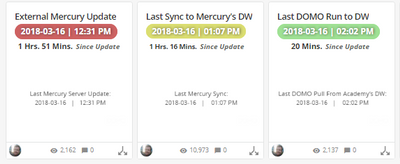# Summary number color rule?

I understand you can be powerful with the summary number in a card, referencing HTML or calculated fields/etc.  Is there a way to set a color rule to it? So if i want to make the summary number RED if below 15, or GREEN if above 50, then i could make the card-size tiny and fit more cards on a page and the end user could just reference what card is RED/YELLOW and click on that card for more information.  Is this possible?

• Hi Greg_B,

you might want to check out this link (especially example 3).

What you will need to do is create a beast mode with CONCAT() and put a CASE WHEN statement into the HTML color rule, so something like

CONCAT('<a style="color: ,

(CASE WHEN `Value` < 15 THEN '#f00'

WHEN > 50 THEN '#00c200'

ELSE '#ff0'

END)

,">',`Value`),'</a>')

HTH JHL

• I use a lot of this in my summary numbers. For Example (see snippet and note, the color rules are applied to all cards: green, yellow, red  - just including the 3 cards together so you can see the different colors).• Green
• Time Since Update < 1 hour
• Hex: #30322F
• Yellow
• Time Since Update: between 1 hour and 1.66 hours
• Hex: #DBDE71
• Red
• Time Since Update: > 1.66 hours
• Hex: #646763

Beast mode is very long, but essentially as follows for green.

case when datediff(minutes,NOW(),[Date])  / 60 < 1

HTML / CSS For Date | Time

then

then CONCAT(
'<b style="
margin-right: -2px;
font-size: .69em;
text-shadow: 1px 0px 8px rgba(50, 50, 50, 0.88);
color: #ffffff;
background-color: #9FE194;
border: 1px solid #9FE194;
,CONCAT(DATE(CONVERT_TZ(MAX(`LastEnteredDateTime`),'US/Mountain','UTC'))
,' | '
,TIME_FORMAT(TIME(CONVERT_TZ(`LastEnteredDateTime`,'US/Mountain','UTC')),'%h:%i %p'))
,'</b>'
,'<br>' - Move Down Line Below

HTML / CSS for Time Since Update (hrs, mins)
,'<b style="
margin: 0px 0px 0px 25px;
font-weight: Bold;
Font-size: .62em;
color: #30322F;">'

Using MOD([Column],1) Spits Out the Decimal (I.E. 10.3432 = MOD(10.3432,1) = .3432) To Get Hrs, Min
,FLOOR(MOD(ABS(((DATEDIFF(DATE(CONVERT_TZ(NOW(),'GMT','US/Mountain')),DATE(CONVERT_TZ(MAX(`LastEnteredDateTime`),'US/Mountain','UTC'))) * 86400)
+
(TIME_TO_SEC(CONVERT_TZ(NOW(),'GMT','US/Mountain')) - TIME_TO_SEC(CONVERT_TZ(MAX(`LastEnteredDateTime`),'US/Mountain','UTC'))))
/
3600),1)*60)
,' '
,'Mins.'
,'</b>'
,' '

HTML / CSS For Wording 'Since Update'
,'<b style="
font-style: italic;
Font-size: .58em;
color: #646763;">'
,'Since Update'
,'</b>'
-- End First Concat ('the then to the case when')

Here I would put my next when datediff(minutes,NOW(),[Date])  / 60 BETWEEN 1 and 1.66

etc. etc.

Hope this helps.

• Hello,

I'm having difficulty with creating this beast mode. I was looking to have a similar rule, where my summary number would be green if above a certain value, and red if below. However, I'm getting an "invalid formula" error when trying this:

CONCAT('<a style="color: ,

(CASE WHEN `Value` < 15 THEN '#f00'

WHEN > 50 THEN '#00c200'

ELSE '#ff0'

END)

,">',`Value`),'</a>')

I replaced the 'Value' portion with the dataset field I wanted, but it wasn't being picked up as an actual value (i.e. it was in red font instead of blue). I'm not sure what I'm missing, so any help would be appreciated.

• Thomas,

Have a look. I bolded the small typo in the beast mode. the original beastmode had " vs. '.

Hope this helps.

CONCAT('<a style='color: ,

(CASE WHEN `Value` < 15 THEN '#f00'

WHEN > 50 THEN '#00c200'

ELSE '#ff0'

END)

,">',`Value`),'</a>')

This discussion has been closed.